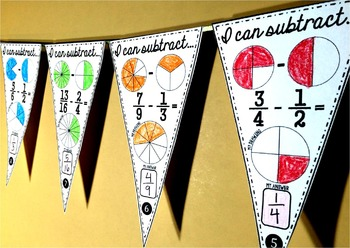# Subtracting Fractions Pennant Activity

Rated 5 out of 5, based on 26 reviews
26 Ratings;
4th - 6th
Subjects
Resource Type
Standards
Formats Included
• PDF
Pages
8 pages
Report this resource to TPT
##### Also included in
1. This bundle includes 6 sets of fractions pennants for comparing, adding, subtracting, multiplying, dividing and converting. Each set includes math pennants for students to color, an optional student answer sheet and answer keys.Math pennants are a fun way to show off student work. Students love seei
Price \$12.50Original Price \$21.00Save \$8.50

### Description

Students subtract fractions in this activity that doubles as classroom decor. Students color partitioned circles to show the given fractions, subtract the fractions, and then show the difference as a picture and as a fraction. All problems have unlike denominators that are related so that only one denominator needs to be changed in order for the two fractions to have a common denominator. Once a pennant is complete, it can be hung along a string in your classroom to show the world that, "Hey, we know how to subtract fractions!"

**Holiday version of this pennant is included in Fraction Ornaments mini-bundle.

Fractions range from halves to sixteenths.

Included are 20 pennants with fraction subtraction problems, an optional student answer sheet and an answer key. Each group of students can be given a set of pennants, crayons, scissors and either glue, tape or a stapler. Students can be in charge of cutting out the pennants. Groups can compete to see which group can complete the most pennants or your entire class can add pennants to one string as they complete each one.

You may also like:

Multiplying Fractions Pennant

Fraction Pennants Mini-Bundle

Total Pages
8 pages
Included
Teaching Duration
N/A
Report this resource to TPT
Reported resources will be reviewed by our team. Report this resource to let us know if this resource violates TPT’s content guidelines.

### Standards

to see state-specific standards (only available in the US).
Understand a fraction 𝘢/𝘣 with 𝘢 > 1 as a sum of fractions 1/𝘣.
Understand addition and subtraction of fractions as joining and separating parts referring to the same whole.
Add and subtract fractions with unlike denominators (including mixed numbers) by replacing given fractions with equivalent fractions in such a way as to produce an equivalent sum or difference of fractions with like denominators. For example, 2/3 + 5/4 = 8/12 + 15/12 = 23/12. (In general, 𝘢/𝘣 + 𝘤/𝘥 = (𝘢𝘥 + 𝘣𝘤)/𝘣𝘥.)